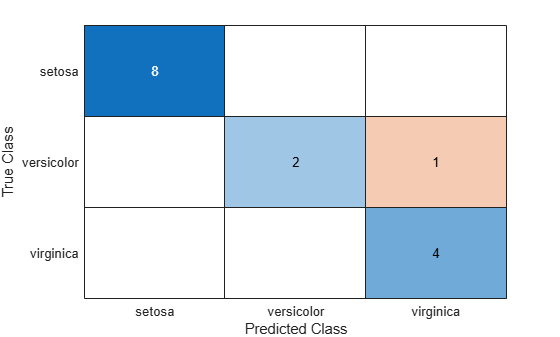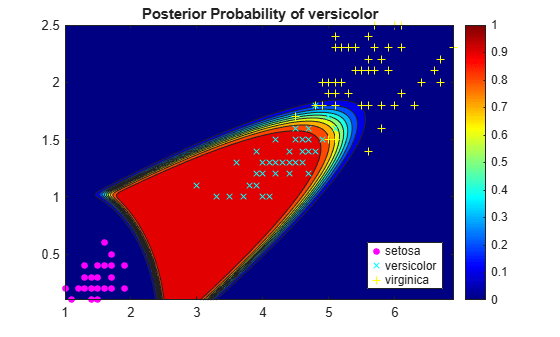predict

Predict labels using discriminant analysis classification model

Description

label = predict(Mdl,X) returns a vector of predicted class labels for the predictor data in the table or matrix X, based on the trained discriminant analysis classification model Mdl.

[label,score,cost] = predict(Mdl,X) also returns:

• A matrix of classification scores (score) indicating the likelihood that a label comes from a particular class. For discriminant analysis, scores are posterior probabilities.

• A matrix of expected classification cost (cost). For each observation in X, the predicted class label corresponds to the minimum expected classification cost among all classes.

Input Arguments

expand all

Discriminant analysis classification model, specified as a ClassificationDiscriminant or CompactClassificationDiscriminant model object returned by fitcdiscr.

Predictor data to be classified, specified as a numeric matrix or table.

Each row of X corresponds to one observation, and each column corresponds to one variable. All predictor variables in X must be numeric vectors.

• For a numeric matrix, the variables that compose the columns of X must have the same order as the predictor variables that trained Mdl.

• For a table:

• predict does not support multicolumn variables and cell arrays other than cell arrays of character vectors.

• If you trained Mdl using a table (for example, Tbl), then all predictor variables in X must have the same variable names and data types as those that trained Mdl (stored in Mdl.PredictorNames). However, the column order of X does not need to correspond to the column order of Tbl. Tbl and X can contain additional variables (response variables, observation weights, etc.), but predict ignores them.

• If you trained Mdl using a numeric matrix, then the predictor names in Mdl.PredictorNames and corresponding predictor variable names in X must be the same. To specify predictor names during training, see the PredictorNames name-value pair argument of fitcdiscr. X can contain additional variables (response variables, observation weights, etc.), but predict ignores them.

Data Types: table | double | single

Output Arguments

expand all

Predicted class labels, returned as a categorical or character array, logical or numeric vector, or cell array of character vectors.

label:

• Is the same data type as the observed class labels (Y) that trained Mdl. (The software treats string arrays as cell arrays of character vectors.)

• Has length equal to the number of rows of X.

Predicted class posterior probabilities, returned as a numeric matrix of size N-by-K. N is the number of observations (rows) in X, and K is the number of classes (in Mdl.ClassNames). score(i,j) is the posterior probability that observation i in X is of class j in Mdl.ClassNames.

Expected classification costs, returned as a matrix of size N-by-K. N is the number of observations (rows) in X, and K is the number of classes (in Mdl.ClassNames). cost(i,j) is the cost of classifying row i of X as class j in Mdl.ClassNames.

Examples

expand all

Load Fisher's iris data set. Determine the sample size.

N = size(meas,1);

Partition the data into training and test sets. Hold out 10% of the data for testing.

rng(1); % For reproducibility
cvp = cvpartition(N,'Holdout',0.1);
idxTrn = training(cvp); % Training set indices
idxTest = test(cvp);    % Test set indices

Store the training data in a table.

tblTrn = array2table(meas(idxTrn,:));
tblTrn.Y = species(idxTrn);

Train a discriminant analysis model using the training set and default options.

Mdl = fitcdiscr(tblTrn,'Y');

Predict labels for the test set. You trained Mdl using a table of data, but you can predict labels using a matrix.

labels = predict(Mdl,meas(idxTest,:));

Construct a confusion matrix for the test set.

confusionchart(species(idxTest),labels)Mdl misclassifies one versicolor iris as virginica in the test set.

Load Fisher's iris data set. Consider training using the petal lengths and widths only.

X = meas(:,3:4);

Train a quadratic discriminant analysis model using the entire data set.

Define a grid of values in the observed predictor space. Predict the posterior probabilities for each instance in the grid.

xMax = max(X);
xMin = min(X);
d = 0.01;
[x1Grid,x2Grid] = meshgrid(xMin(1):d:xMax(1),xMin(2):d:xMax(2));

[~,score] = predict(Mdl,[x1Grid(:),x2Grid(:)]);
Mdl.ClassNames
ans = 3x1 cell
{'setosa'    }
{'versicolor'}
{'virginica' }

score is a matrix of class posterior probabilities. The columns correspond to the classes in Mdl.ClassNames. For example, score(j,1) is the posterior probability that observation j is a setosa iris.

Plot the posterior probability of versicolor classification for each observation in the grid and plot the training data.

figure;
contourf(x1Grid,x2Grid,reshape(score(:,2),size(x1Grid,1),size(x1Grid,2)));
h = colorbar;
caxis([0 1]);
colormap jet;
hold on
gscatter(X(:,1),X(:,2),species,'mcy','.x+');
axis tight
title('Posterior Probability of versicolor');
hold offThe posterior probability region exposes a portion of the decision boundary.

expand all